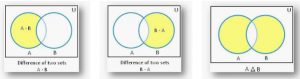SET THEORY

Set is a collection of well defined and distinct objects.

POINTS TO KNOW

• n(AᴜB) is the number of elements present in either of the sets A or B.
• n(A∩B) is the number of elements present in both the sets A and B.

FORMULAS

For two sets A and B

• n(AᴜB) = n(A) + (n(B) – n(A∩B)

For three sets A, B and C

• n(AᴜBᴜC) = n(A) + n(B) + n(C) – n(A∩B) – n(B∩C) – n(C∩A) + n(A∩B∩C)

SOME VEEN DI AGRAM REPRESENTATIONS:

In above diagram  set  A is the subset of set B